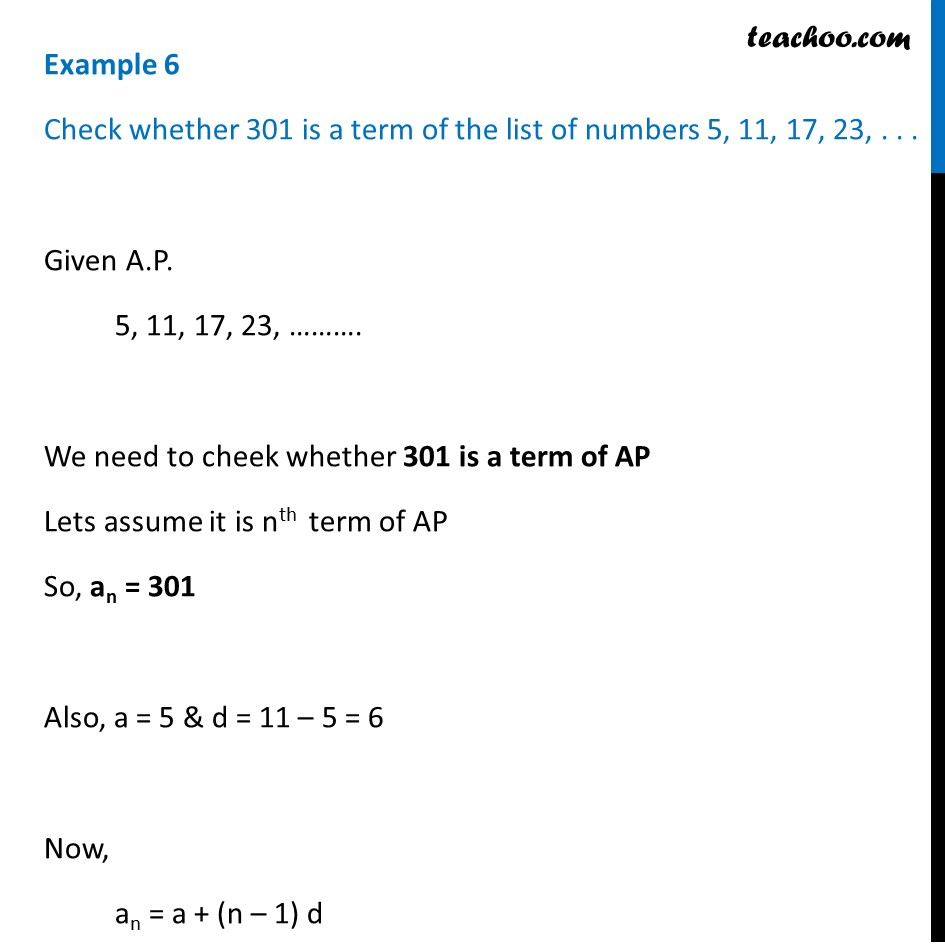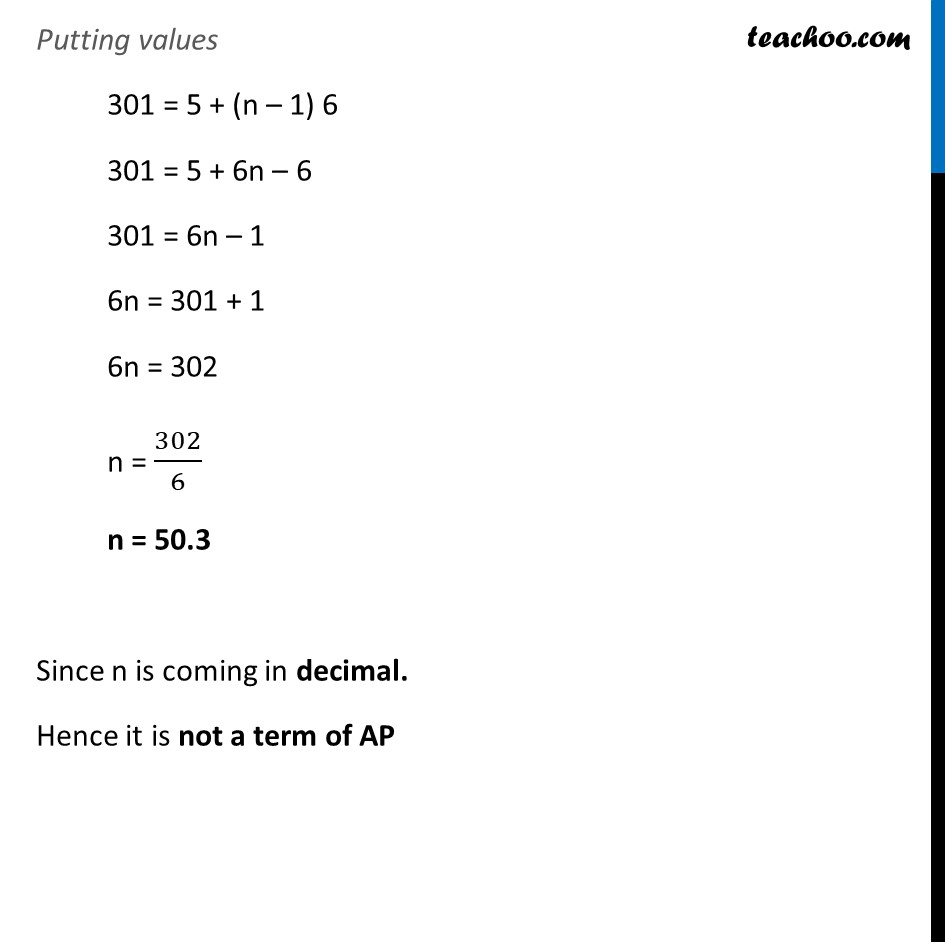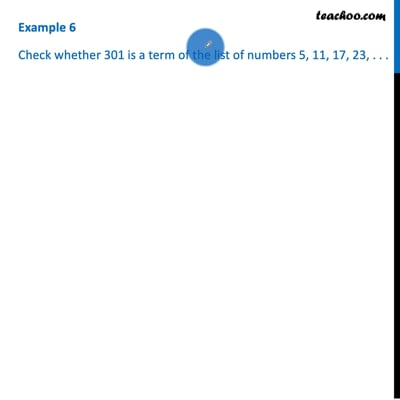Finding n

Chapter 5 Class 10 Arithmetic Progressions (Term 2)
Concept wiseThis video is only available for Teachoo black users

### Transcript

Example 6 Check whether 301 is a term of the list of numbers 5, 11, 17, 23, . . . Given A.P. 5, 11, 17, 23, ………. We need to cheek whether 301 is a term of AP Lets assume it is nth term of AP So, an = 301 Also, a = 5 & d = 11 – 5 = 6 Now, an = a + (n – 1) d Putting values 301 = 5 + (n – 1) 6 301 = 5 + 6n – 6 301 = 6n – 1 6n = 301 + 1 6n = 302 n = 302/6 n = 50.3 Since n is coming in decimal. Hence it is not a term of AP